# Division Worksheets

#### How to Divide Numbers

Among the basic arithmetic operations, the division is the hardest one. Students find it difficult to solve division questions. The major reason for this is the lack of understanding and concepts at the initial stages. So, today, we'll provide you with some basic steps which can help you divide numbers easily. 1. Keep in mind that we must start dividing them from the first place of the given number. 2. We must also ensure that the divisor must be smaller than the dividend. 3. Subtract the product from the dividend and then take the next number until it is finished. 4. Quotient must always be written above the line and product below the dividend.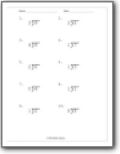###### Single Digit Division

These problems are setup horizontal found within a set quotient bracket for your to work off of.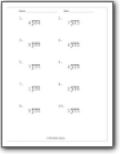###### Single Into Double Digit

You are provided with a quotient bracket that contains a double digit value to be divided by a single digit number.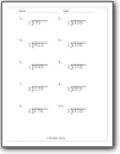###### Fixed Divisor

A triple digit value is found with a quotient bracket and you will work five problems that contain the same fixed divisor.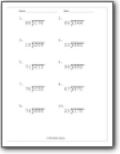###### Triple Divided By Double Digits

You will use the quotient brackets to divide a double digit value into a triple digit value.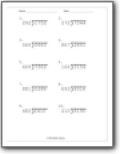###### Triple and Quadruple Digit Division

A quadruple digit value is found contained with quotient brackets and you are to divide it by a triple digit value.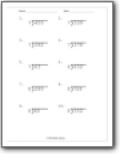###### Single Digit Into Triple Digit Division

A single digit value is located outside of the quotient bracket for you to process the division of the triple digit value.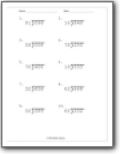###### Double Into Triple Digit Division

Find the quotient of two-digit into the three-digit values.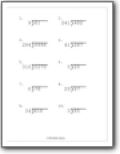###### Division Review

All the problems vary by the number of digit values that are included within the quotient bracket.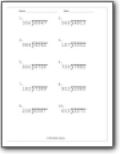###### Three Into Four Digit Division

These problems require you to pay particular attention to place value, along the way.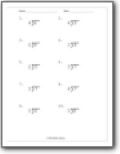###### Simple Division with Remainders

These are simple single digit problems that all result in remainders.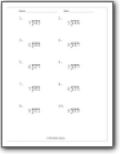###### Single Into Double Digits w/ Remainders

We step up the concept of remainders to a new level.